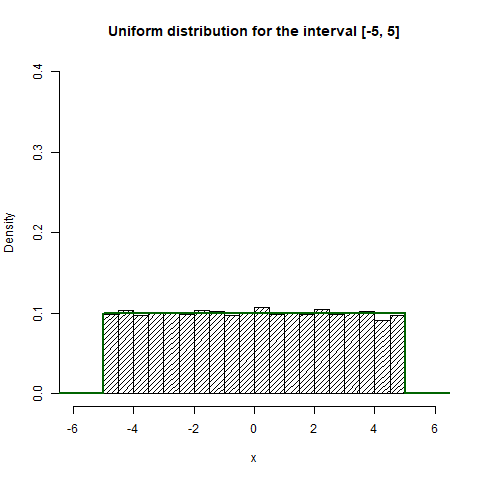# Create Random Deviates of Uniform Distribution in R Programming – runif() Function

• Last Updated : 16 Aug, 2021

runif() function in R Language is used to create random deviates of the uniform distribution.

Syntax: runif(n, min, max)
Parameters:
n: represents number of observations
min, max: represents lower and upper limits of the distribution

Example 1:

## r

 `# Create uniform distribution between range -1 to 1``x <- ``runif``(20, min = -1, max = 1)` `# Print distribution``print``(x)`

#### Output:

```   0.90453974 -0.97083088  0.83531295  0.19008941  0.52278078 -0.75730955
  0.21659920 -0.26621211  0.11967620  0.42081991  0.75518470 -0.41519833
 -0.03734007  0.57365077  0.76156708  0.97314141 -0.41957919 -0.08910355
  0.29047038 -0.67069856```

Example 2:

## r

 `# Create uniform distribution of range -5 to 5``unif <- ``runif``(10000, min = -5, max = 5)` `# Output to be present as PNG file``png``(file = ``"runifGFG.png"``)` `# Create Histogram``hist``(unif, freq = ``FALSE``,``     ``xlab = ``'x'``, ``     ``ylim = ``c``(0, 0.4),``     ``xlim = ``c``(-6, 6),``     ``density = 20,``     ``main = ``"Uniform distribution for the interval [-5, 5]"``)` `# Show density of distribution using curve``curve``(``dunif``(x, min = -5, max = 5),``      ``from = -10, to = 10,``      ``n = 100000,``      ``col = ``"darkgreen"``,``      ``lwd = 2,``      ``add = ``TRUE``,``      ``yaxt = ``"n"``,``      ``ylab = ``'probability'``)` `# Saving the file``dev.off``()`

Output:My Personal Notes arrow_drop_up